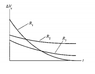# Drawing a Circuit from Voltage-Time Graph

• friendbobbiny

## Homework Statement

The attached graph shows the relationship between time and voltage for three resistors. A capacitor is discharging current through three "separate" resistors. How resistors might be positioned in a circuit to give rise to the graphs.

## Homework Equations

V = IR
CR = $$\uptau$$

## The Attempt at a Solution

Separate cannot mean in parallel, or else the graphs would all begin at the same voltage.

My attempt to prove that
"Separate cannot mean completely in series either" ended in squash:

The resistance order can be shown to be: R2>R3>R1

RC = Tau gives the time constant for a capacitor. The greater tau is, the longer it takes a capacitor discharge or acquire charge. The resistors with the greater resistances, consequently, are the ones that takes the longer to reach V = 0.

What approach can I use?

I take it we are supposed to guess what the graphs look like?

Sorry for forgetting to attach the graph:

nah, I wanted to determine what the circuit woud look like.

#### Attachments

•Screen Shot 2015-01-11 at 10.34.07 AM.png
1.7 KB · Views: 706
Sorry, my original post wasn't clear

1. Homework Statement

The attached graph shows the relationship between time and voltage for three resistors. A capacitor is discharging current through three "separate" resistors. How can resistors be positioned in a circuit so that the graph describes them?

## Homework Equations

V = IR
CR = $$\uptau$$

## The Attempt at a Solution

Separate cannot mean in parallel, or else the graphs would all begin at the same voltage.

My attempt to prove that
"Separate cannot mean completely in series either" ended in squash:

The resistance order can be shown to be: R2>R3>R1

RC = Tau gives the time constant for a capacitor. The greater tau is, the longer it takes a capacitor discharge or acquire charge. The resistors with the greater resistances, consequently, are the ones that takes the longer to reach V = 0.

What approach can I use?

Yes, but the point is that there are obviously three SEPARATE circuit layouts to get the three different graphs. What they are, based on the condition that each use exactly 3 resistors, is obvious. What do you think they might be?

three SEPARATE circuit layouts to get the three different graphs

Ah so I misunderstood the problem? I need to identfy three circuit layouts that each use three resistors.

If I understand the problem, then the approach should be easy:

We already concluded that R2>R3>R1, where R2,R3 and R1 are presumably the net resistances that combinations of the three resistors create.

The component resistors that comprise these net resistors can be assumed to be the same, without loss of generality:

so that R2 has: three Rx resistors in series
R3 has: 2 Rx in series and one in parallel
R1 has: three in parallel

Other layouts would work.

Yep. That's what I came up with.

If that's the accepted answer then you have got off lightly. :)

I would have said the plot with R2 is definitely never going to reach anywhere near zero. That for R1 does look like levelling out on the x axis. But unless I'm mistaken, that exponential plot for R3 looks like it's headed below the x axis.